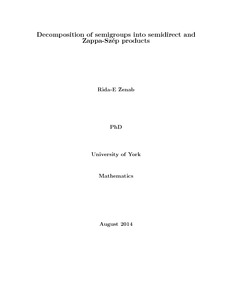# Decomposition of semigroups into semidirect and Zappa-Sz\'{e}p products

Zenab, Rida-E (2014) Decomposition of semigroups into semidirect and Zappa-Sz\'{e}p products. PhD thesis, University of York.Preview
Text
__userfs_rzz500_w2k_Desktop_RidaThesisfinal.pdf
This thesis focuses on semidirect and Zappa-Sz\'{e}p products in the context of semigroups and monoids. We present a survey of direct, semidirect and Zappa-Sz\'{e}p products and discuss correspondence between external and internal versions of these products for semigroups and monoids. Particular attention in this thesis is paid to a wide class of semigroups known as restriction semigroups. We consider Zappa-Sz\'{e}p product of a left restriction semigroup $S$ with semilattice of projections $E$ and determine algebraic properties of it. We prove that analogues of Green's lemmas and Green's theorem hold for certain semigroups where Green's relations $\ar,\el,\eh$ and $\dee$ are replaced by $\art_E,\elt_E, \eht_E$ and $\adt_E$. We show that if $\eht_E$ is a congruence on a certain semigroup $S$, then any right congruence on the submonoid $\wt H^e_E$ (the $\eht_E$-class of $e$), where $e\in E$, can be extended to a congruence on $S$. We introduce the idea of an {\em inverse skeleton} $U$ of a semigroup $S$ and examine some conditions under which we obtain skeletons from monoids. We focus on a result of Kunze \cite{kunze:1983} for the Bruck-Reilly extension $\mbox{BR}(M,\theta)$ of a monoid $M$, showing that $\mbox{BR}(M,\theta)$ is a Zappa-Sz\'{e}p product of $\N^0$ under addition and a semidirect product $M\rtimes \N^0$. We put Kunze's result in more general framework and give an analogous result for certain restriction monoids. We consider the {\it $\lambda$-semidirect product} of two left restriction semigroups and prove that it is left restriction. In the two sided case using the notion of double action we prove that the $\lambda$-semidirect product of two restriction semigroups is restriction. introduce the notion of $(A,T)$-propernessto prove the results analogous to McAlister's covering theorem and O'Carroll's embedding theorem for monoids and left restriction monoids under some conditions. We extend the notion of the $\lambda$-semidirect product of two restriction semigroups $S$ and $T$ to develop $\lambda$-Zappa-Sz\'{e}p products and construct a category. In the special case where $S$ is a semilattice and $T$ is a monoid we order our category to become inductive and thus obtain a restriction semigroup via the use of the standard pseudo-product.